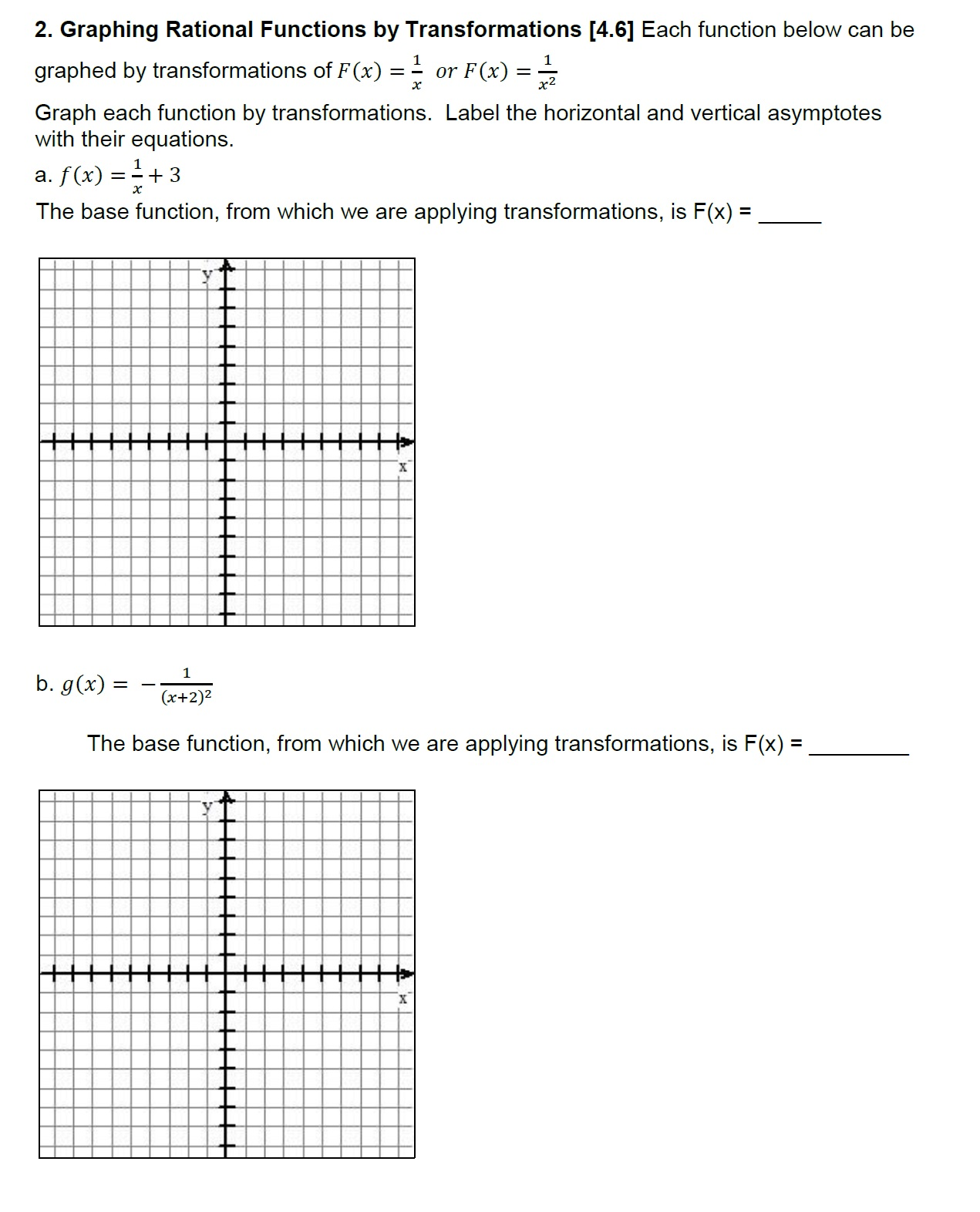# 2. Graphing Rational Functions by Transformations [4.6] Each function below can begraphed by transformations of F(x) or F(x)Graph each function by transformations. Label the horizontal and vertical asymptoteswith their equations.a, f(x) =-+ 3The base function, from which we are applying transformations, is F(x) -(x+2)2The base function, from which we are applying transformations, is F(x) -

Question
6 viewshelp_outlineImage Transcriptionclose2. Graphing Rational Functions by Transformations [4.6] Each function below can be graphed by transformations of F(x) or F(x) Graph each function by transformations. Label the horizontal and vertical asymptotes with their equations. a, f(x) =-+ 3 The base function, from which we are applying transformations, is F(x) - (x+2)2 The base function, from which we are applying transformations, is F(x) - fullscreen
check_circle

Step 1

Graphing the given function by applying transformations on a simpler function

Step 2

(a)

Step 3

The last equation shows that the graph of y=f(x) is obtained from the graph of y=F(x)=1/x...

### Want to see the full answer?

See Solution

#### Want to see this answer and more?

Solutions are written by subject experts who are available 24/7. Questions are typically answered within 1 hour.*

See Solution
*Response times may vary by subject and question.
Tagged in

### Algebra